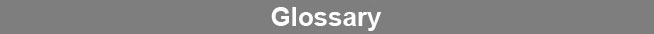Select Page### Beer-Lambert Law

The Beer-Lambert Law is an equation that describes the linear relationship between the absorption and the concentration of the absorbing material (absorber). The law states that absorbance (ABS) is equal to absorptivity a multiplied by the path length or the distance the light travels through the sample b, multiplied by the concentration c (ABS = abc).www.brendan.com